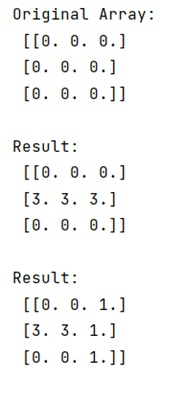# What does [:, :] mean on NumPy arrays?

Learn about the meaning of [:, :] in NumPy arrays.
Submitted by Pranit Sharma, on February 28, 2023

NumPy is an abbreviated form of Numerical Python. It is used for different types of scientific operations in python. Numpy is a vast library in python which is used for almost every kind of scientific or mathematical operation. It is itself an array which is a collection of various methods and functions for processing the arrays.

## Use of [:, :] in NumPy Arrays

Basically, [: , :] stands for everything from the beginning to the end just like for lists. The first colon stands for the first dimension and the second colon is for the second dimension.

If we use the second colon alone and specify a value for the first colon, it will work for all the rows but only for the specific column.

If we use the first colon alone and specify a value for the second colon, it will work for all the columns but only for the specific row.

Let us understand with the help of an example,

## Python code to demonstrate the use of [:, :] in NumPy arrays

```# Import numpy
import numpy as np

# Creating a numpy array
arr = np.zeros((3, 3))

# Display original image
print("Original Array:\n",arr,"\n")

# working on all rows but a specific column
arr[1, :] = 3

# Display result
print("Result:\n",arr,"\n")

# working on all columns but a specific row
arr[:, 2] = 1

# Display result
print("Result:\n",arr,"\n")
```

Output:Languages: » C » C++ » C++ STL » Java » Data Structure » C#.Net » Android » Kotlin » SQL
Web Technologies: » PHP » Python » JavaScript » CSS » Ajax » Node.js » Web programming/HTML
Solved programs: » C » C++ » DS » Java » C#
Aptitude que. & ans.: » C » C++ » Java » DBMS
Interview que. & ans.: » C » Embedded C » Java » SEO » HR
CS Subjects: » CS Basics » O.S. » Networks » DBMS » Embedded Systems » Cloud Computing
» Machine learning » CS Organizations » Linux » DOS
More: » Articles » Puzzles » News/Updates